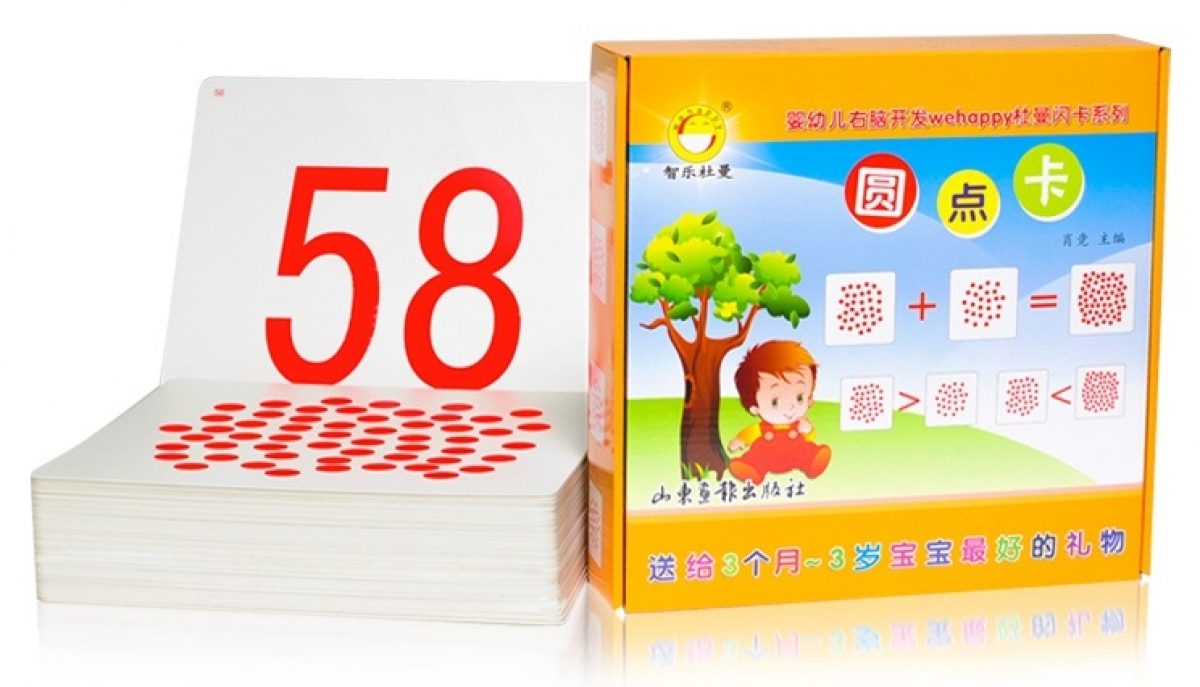# Math Dot Red Flash Card

RM 109.00

Math Dot Red Flash Card

Type A :
Dot Red Card
Total of 111pcs
Size 28 x 28cm
Paper Quality of 450g
Weight 4.3kg

Dot Red 0 to 100, 200, 300 total of 102pcs
Mathematic symbol - Addition, Subtraction, Division, Multiplication, Equal, Not equal, Bigger or Smaller, "Y" Total of 8 cards

...........

Type B:
Pattern Dot Card
Total of 129cards
Size 25 x 25cm
Paper Quality of 400g
Weight 4kg

Dot Red Card 0 to 20, 50, 100 total of 23 cards
Mathematic symbol - Addition, Subtraction, Division, Multiplication, Equal, Bigger or Smaller, Total of 6 cards
1 to 100 with 20 different patterns total of 100 cards